# strcmp

Compare strings

## Syntax

``tf = strcmp(s1,s2)``
``tf = strcmp(s1,s2,n)``

## Description

example

````tf = strcmp(s1,s2)` compares strings `s1` and `s2`. When using MATLAB® as the action language, `strcmp` returns ```1 (true)``` if the two strings are identical. Otherwise, `strcmp` returns `0 (false)`. When using C as the action language, `strcmp` returns `0` if the two strings are identical. Otherwise, `strcmp` returns a nonzero integer where: The sign of the output value depends on the lexicographic order of the input strings `s1` and `s2`.The magnitude of the output value depends on the compiler that you use. This value can differ in simulation and generated code. Strings are considered identical when they have the same size and content. `strcmp` is case sensitive.```

example

````tf = strcmp(s1,s2,n)` returns `0` if the first `n` characters in `s1` and `s2` are identical in charts that use C as the action language. For this functionality when using MATLAB as the action language, see strncmp.```

## Examples

expand all

Return a value of `1(true)` if the strings are equal.

`tf = strcmp("abc","abc");`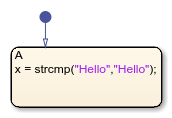Return a `0(false)` value if the strings are not equal.

`tf = strcmp("abc","abcd");`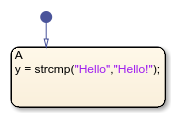You can compare and sort string with relational operators.

Use `==` to determine which elements of two string are equal. This is an alternative way to execute ```strcmp("abc", "abc") == 1```.

`"abc" == "abc"`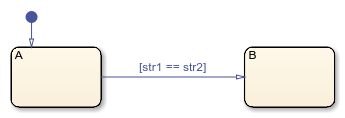Use `~=` to determine which elements of two string are not equal. This is an alternative way to execute ```strcmp("abc", "abc") ~= 1```.

`"abc" ~= "abc"`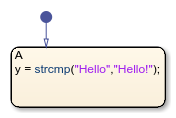Return a value of `0` if the strings are equal.

`tf = strcmp("abc","abc");`Return a nonzero value if the strings are not equal.

`tf = strcmp("abc","abcd");`Use `==` to determine which elements of two strings are equal. This is an alternative way to execute ```strcmp("abc", "abc") == 1```.

`"abc" == "abc"`Use `~=` to determine which elements of two strings are not equal. This is an alternative way to execute ```strcmp("abc", "abc") != 1```.

`"abc" != "abc"`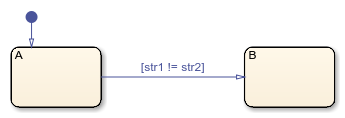Return a value of `0` if the substrings are equal in charts that use C as the action language. To use this functionality when using MATLAB as the action language, see strncmp.

`tf = strcmp("abc","abcd",3);`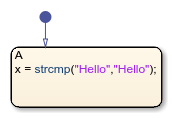### Topics

Introduced in R2018b

## SupportGet trial now